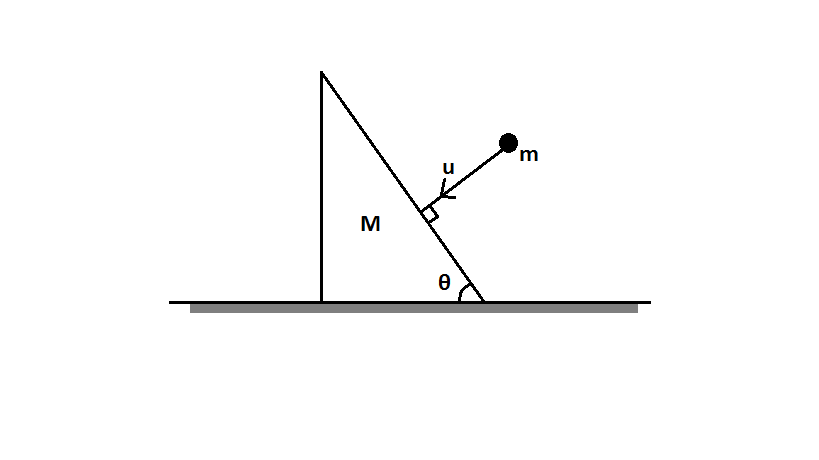# Problems with wedges (1)In the figure shown above, a ball of mass $m$ collides perpendicularly with constant velocity $u$ on a smooth stationary wedge of mass $M$ with inclination $\theta$ kept on a smooth horizontal plane. If the coefficient of restitution of collision is $e$, then determine the velocity of the wedge after collision.

Details:

• Assume gravity has no effect on the ball of mass $m$ until it collides with the wedge and during its collision.

×